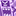Announcements
Applications are open for the 2024 Qlik Luminary Program. Apply by December 15 here.
cancel
Showing results for
Did you mean:Contributor II

## how to color subtotals in pivot table

hi,

Need some help to color subtotals in pivot table.

In my Pivot table  i have 2 dimensions as rows and 1 dimension as column  and 1 measure .

my measure is Count({<[WeekSerial]={">\$(=max(WeekSerial)-6)<=\$(=max(WeekSerial))"}>} [Office])

my Pivot table look like this:now i am coloring the totals with the the expression If(Dimensionality() = 0, RGB(203,209,225)) to color the totals row and it is working and now i want to color the subtotals in the above table. Can anyone help me how can i achieve this?

1 Solution

Accepted SolutionsMVP

Hi @Rama1

Try with Rowno() function like below.

=If(Dimensionality() = 0, RGB(203,209,225), If(RowNo()=0, Red()))

Hope it helps

Thanks & Regards, Mayil Vahanan R
4 RepliesMVP

Hi @Rama1

Try with Rowno() function like below.

=If(Dimensionality() = 0, RGB(203,209,225), If(RowNo()=0, Red()))

Hope it helps

Thanks & Regards, Mayil Vahanan RContributor II
Author

Thank you so much for your response @MayilVahanan .

and i achieved what i want with the help of your solution, but is there any way to color only subtotals in the above table without background color along with my expression(this expression i need to color the totals with background color)

=If(Dimensionality() = 0, RGB(203,209,225)

RamaContributor II
Author

hi, i achieved what i want. Thank you so muchContributor II

Hi,

how did you achieve to color only the total rows without the other rows? I've been looking for this function for a long time.Tags
Community Browser